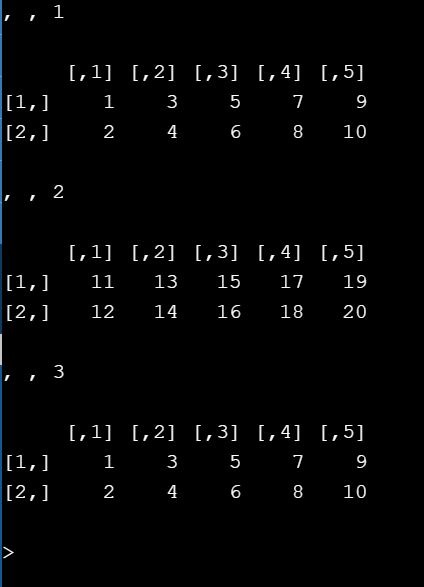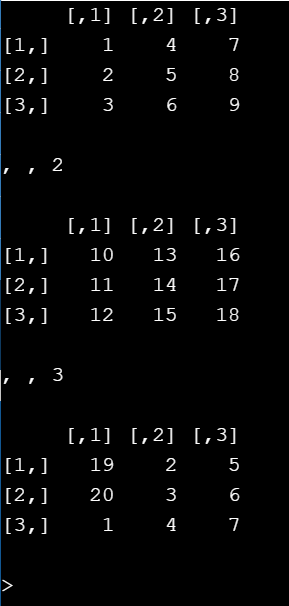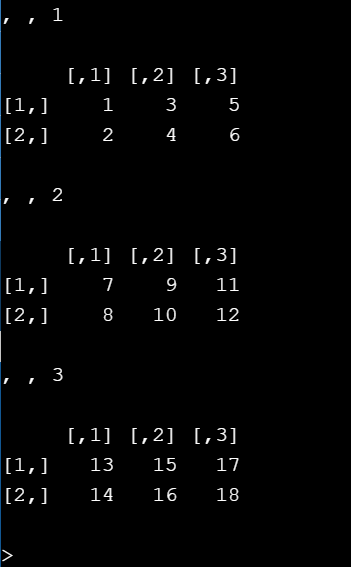Related Articles

# How to create an array from vectors in R ?

• Last Updated : 24 Jun, 2021

In this article, we will discuss how to create an array from the vectors in R Programming Language. We can create an array using array() function. We have to pass the vectors and dim() as parameters. Here dim() function is used to give dimensions for the array.

Syntax:

array_name = array( c(vector 1 , vector 2 , . . . , vector n)  , dim=c( no.of rows , no.of columns  , no.of arrays ))

The approach is straightforward first we need to create vectors and then pass them to the array() function to create one. Implementation is given below:

Example 1 :

## R

 `# creating the vector with``# 1 to 5 values``vec1=``c``(1:5)` `# creating the vector with``# 6 to 10 values``vec2=``c``(6:10)` `# passing the vectors as parameters``# intp array() function``arr=``array``(``c``(vec1,vec2),dim=``c``(2,5,3))` `# printing the array``print``(arr)`

Output :Example 2 :

## R

 `# creating vector with 1``# to 10 values``vec1=``c``(1:10)` `# creating vector with 11``# to 20 values``vec2=``c``(11:20)` `# passing vectors intp the``# array() function .``arr=``array``(``c``(vec1,vec2),dim=``c``(3,3,3))` `# printing the array``print``(arr)`

Output :Example 3 :

## R

 `# creating vector with 1``# to 9 values``vec1=``c``(1:9)` `# creating vector with 11``# to 27 values``vec2=``c``(10:27)` `# passing the two vectors into``# array() function``arr=``array``(``c``(vec1,vec2),dim=``c``(2,3,3))` `# printing the array``print``(arr)`

Output :My Personal Notes arrow_drop_up